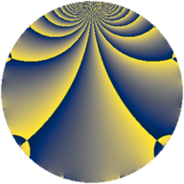# Properties

 Label 546.2.eLevel $546$ Weight $2$ Character orbit 546.e Rep. character $\chi_{546}(545,\cdot)$ Character field $\Q$ Dimension $40$ Newform subspaces $8$ Sturm bound $224$ Trace bound $9$

# Learn more

## Defining parameters

 Level: $$N$$ $$=$$ $$546 = 2 \cdot 3 \cdot 7 \cdot 13$$ Weight: $$k$$ $$=$$ $$2$$ Character orbit: $$[\chi]$$ $$=$$ 546.e (of order $$2$$ and degree $$1$$) Character conductor: $$\operatorname{cond}(\chi)$$ $$=$$ $$273$$ Character field: $$\Q$$ Newform subspaces: $$8$$ Sturm bound: $$224$$ Trace bound: $$9$$ Distinguishing $$T_p$$: $$5$$, $$17$$, $$19$$, $$71$$

## Dimensions

The following table gives the dimensions of various subspaces of $$M_{2}(546, [\chi])$$.

Total New Old
Modular forms 120 40 80
Cusp forms 104 40 64
Eisenstein series 16 0 16

## Trace form

 $$40q + 40q^{4} - 8q^{9} + O(q^{10})$$ $$40q + 40q^{4} - 8q^{9} + 40q^{16} - 56q^{25} + 4q^{30} - 8q^{36} + 12q^{39} + 14q^{42} - 48q^{43} + 28q^{49} - 12q^{51} + 40q^{64} - 4q^{78} - 120q^{79} + 16q^{81} - 36q^{91} + O(q^{100})$$

## Decomposition of $$S_{2}^{\mathrm{new}}(546, [\chi])$$ into newform subspaces

Label Dim. $$A$$ Field CM Traces $q$-expansion
$$a_2$$ $$a_3$$ $$a_5$$ $$a_7$$
546.2.e.a $$2$$ $$4.360$$ $$\Q(\sqrt{-3})$$ None $$-2$$ $$-3$$ $$0$$ $$-5$$ $$q-q^{2}+(-1-\zeta_{6})q^{3}+q^{4}+(-1+2\zeta_{6})q^{5}+\cdots$$
546.2.e.b $$2$$ $$4.360$$ $$\Q(\sqrt{-3})$$ None $$-2$$ $$3$$ $$0$$ $$5$$ $$q-q^{2}+(1+\zeta_{6})q^{3}+q^{4}+(1-2\zeta_{6})q^{5}+\cdots$$
546.2.e.c $$2$$ $$4.360$$ $$\Q(\sqrt{-3})$$ None $$2$$ $$-3$$ $$0$$ $$5$$ $$q+q^{2}+(-1-\zeta_{6})q^{3}+q^{4}+(1-2\zeta_{6})q^{5}+\cdots$$
546.2.e.d $$2$$ $$4.360$$ $$\Q(\sqrt{-3})$$ None $$2$$ $$3$$ $$0$$ $$-5$$ $$q+q^{2}+(1+\zeta_{6})q^{3}+q^{4}+(-1+2\zeta_{6})q^{5}+\cdots$$
546.2.e.e $$8$$ $$4.360$$ 8.0.303595776.1 None $$-8$$ $$0$$ $$0$$ $$0$$ $$q-q^{2}+\beta _{1}q^{3}+q^{4}+(\beta _{1}-\beta _{5}+\beta _{7})q^{5}+\cdots$$
546.2.e.f $$8$$ $$4.360$$ 8.0.$$\cdots$$.11 None $$-8$$ $$0$$ $$0$$ $$0$$ $$q-q^{2}-\beta _{7}q^{3}+q^{4}+(\beta _{1}+\beta _{7})q^{5}+\cdots$$
546.2.e.g $$8$$ $$4.360$$ 8.0.303595776.1 None $$8$$ $$0$$ $$0$$ $$0$$ $$q+q^{2}+\beta _{1}q^{3}+q^{4}+(-\beta _{1}+\beta _{5}-\beta _{7})q^{5}+\cdots$$
546.2.e.h $$8$$ $$4.360$$ 8.0.$$\cdots$$.11 None $$8$$ $$0$$ $$0$$ $$0$$ $$q+q^{2}+\beta _{1}q^{3}+q^{4}+(\beta _{1}+\beta _{7})q^{5}+\cdots$$

## Decomposition of $$S_{2}^{\mathrm{old}}(546, [\chi])$$ into lower level spaces

$$S_{2}^{\mathrm{old}}(546, [\chi]) \cong$$ $$S_{2}^{\mathrm{new}}(273, [\chi])$$$$^{\oplus 2}$$